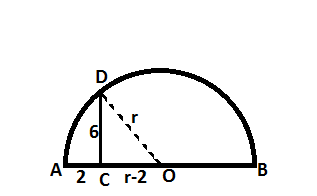Question 21

# A semi-circle is drawn with AB as its diameter. From C, a point on AB, a line perpendicular to AB is drawn meeting the circumference of the semi-circle at D. Given that AC = 2 cm and CD = 6 cm, the area of the semi-circle (in sq. cm) will be:

SolutionIn triangle CDO, which is a right anged triangle, we can use pythagoras theorem.

$$6^2$$ + $$(r-2)^2$$ = $$r^2$$

=> 36 + $$r^2 - 4r + 4$$ = $$r^2$$

=> 4r = 40

=> r = 10

=> Area = $$\pi *10*10/2$$ = $$50\pi$$